Welcome to the Beta version of the Spirax Sarco website intended for final customer testing. During this beta phase, we will be fine tuning the website before the launch of the new website.

We use cookies to give you the best experience on our website. If you continue without changing your cookie settings, we assume that you consent to our use of cookies on this device. You can change your cookie settings at any time but if you do, you may lose some functionality on our website. More information can be found in our Privacy Policy.

Steam Engineering Principles and Heat Transfer

Engineering Units

An overview of the units of measurement used in the Steam and Condensate Loop including temperature, pressure, density, volume, heat, work and energy.

Throughout the engineering industries, many different definitions and units have been proposed and used for mechanical and thermal properties.

The problems this caused led to the development of an agreed international system of units (or SI units: Système International d’Unités). In the SI system there are seven well-defined base units from which the units of other properties can be derived, and these will be used throughout this publication.

The SI base units include length (in metres), mass (in kilograms), time (in seconds) and temperature (in kelvin). The first three will hopefully need no further explanation, while the latter will be discussed in more detail later.

The other SI base units are electric current (in amperes), amount of substance (in moles) and luminous intensity (in candela). These may be familiar to readers with a background in electronics, chemistry and physics respectively, but have little relevance to steam engineering nor the contents of The Steam and Condensate Loop.

Table 2.1.1 shows the derived units that are relevant to this subject, all of which should be familiar to those with any general engineering background. These quantities have all been assigned special names after famous pioneers in the development of science and engineering.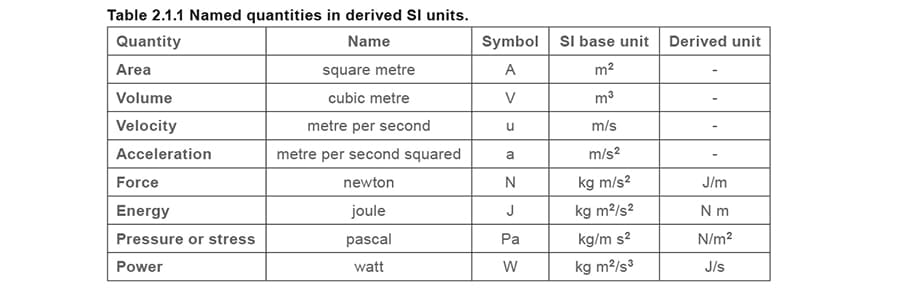There are many other quantities that have been derived from SI base units, which will also be of significance to anyone involved in steam engineering. These are provided in Table 2.1.2.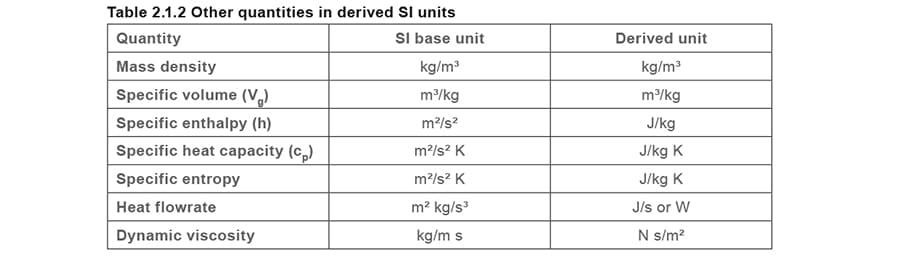Dot notation

• This convention is used to identify a compound unit incorporating rate, for example:
• m = Mass (e.g. kg)
• ṁ = Mass flow per time unit (e.g. kg/h) = Mass flowrate

Multiples and submultiples

Table 2.1.3 gives the SI prefixes that are used to form decimal multiples and submultiples of SI units. They allow very large or very small numerical values to be avoided. A prefix attaches directly to the name of a unit, and a prefix symbol attaches directly to the symbol for a unit.

In summary: one thousand metres may be shown as 1 km, 1 000 m or 10³ m.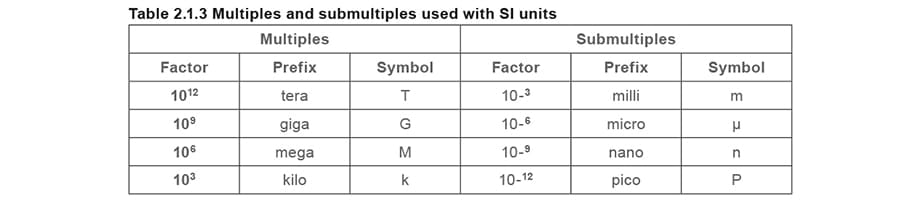Special abbreviations used in steam flowmetering applications

For historical reasons, International Standard ISO 5167 (supersedes BS 1042) which refers to flowmetering, use the following abbreviations in Table 2.1.4.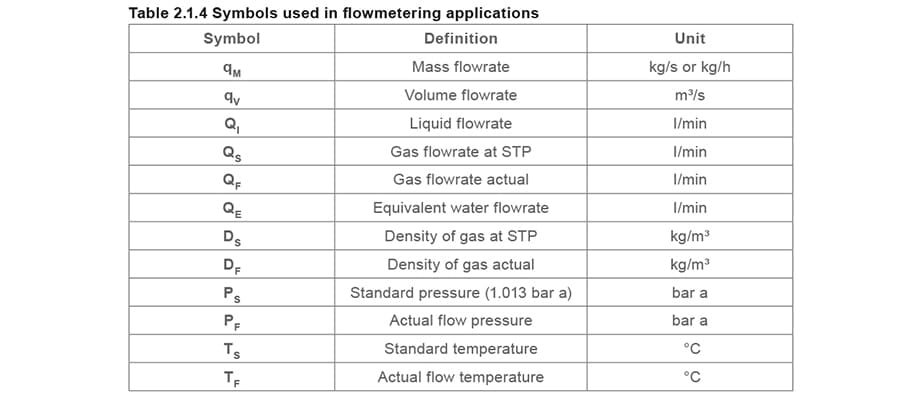STP - Standard temperature and pressure

These are the standard conditions for measurement of the properties of matter. The standard temperature is the freezing point of pure water, 0 °C or 273.16 °K. The standard pressure is the pressure exerted by a column of mercury (symbol Hg) 760 mm high, often designated 760 mm Hg. This pressure is also called one atmosphere and is equal to 1.01325 x 106 dynes per square centimetre, or approximately 14.7 lb per square inch. The density (mass per volume) of a gas is usually reported as its value at STP. Properties that cannot be measured at STP are measured under other conditions; usually the values obtained are then mathematically extrapolated to their values at STP

Symbols

Table 2.1.5 shows the symbols and typical units used in The Steam and Condensate Loop.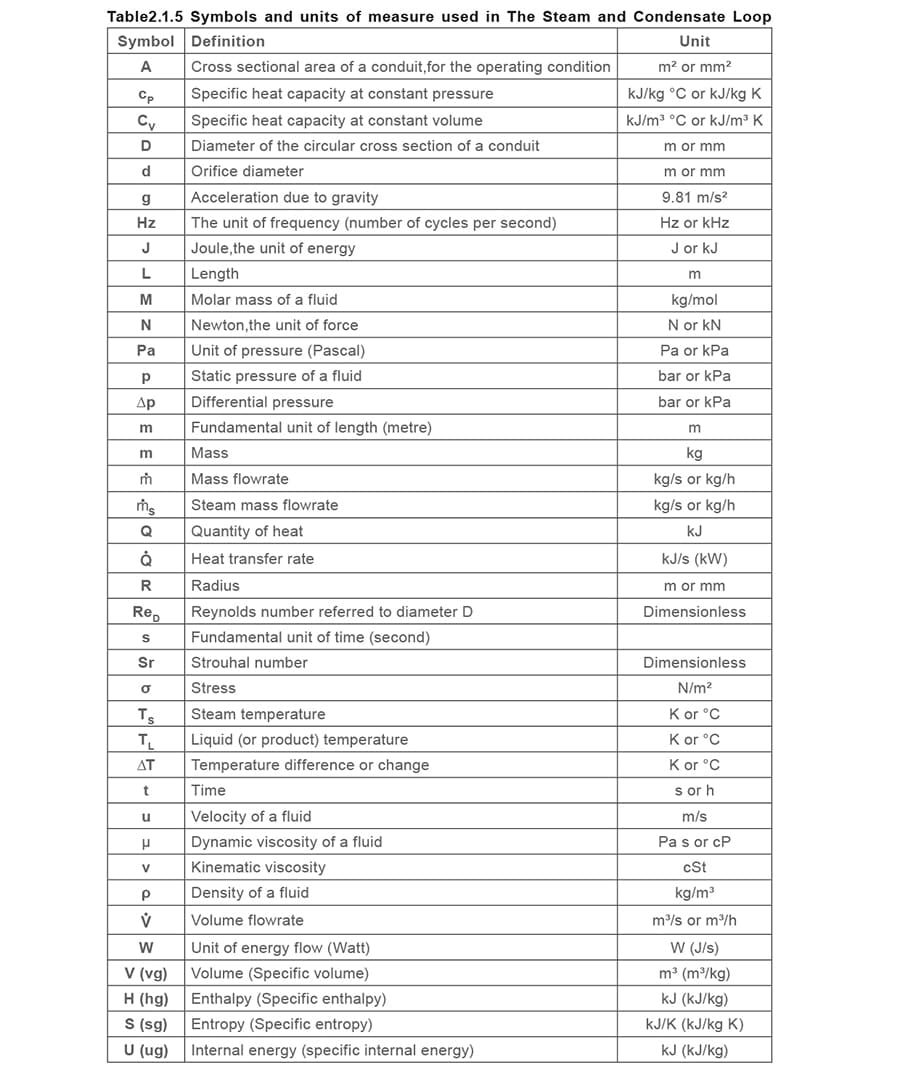Subscripts used with prop​erties​

When using enthalpy, entropy and internal energy, subscripts as shown below are used to identify the phase, for example:

• Subscript f = Fluid or liquid state, for example hf: liquid enthalpy
• Subscript fg = Change of state liquid to gas, for example hfg: enthalpy of evaporation
• Subscript g = Total, for example hg: total enthalpy

Note that, by convention, the total heat in superheated steam is signified by h.

It is also usual, by convention, to signify sample quantities in capital letters, whilst unit quantities are signified in lower case letters.

For example:

Total enthalpy in a sample of superheated steam H kJ

Specific enthalpy of superheated steam h kJ/kg

Temper​ature

The temperature scale is used as an indicator of thermal equilibrium, in the sense that any two systems in contact with each other with the same value are in thermal equilibrium.

The Celsius (°C) scale

This is the scale most commonly used by the engineer, as it has a convenient (but arbitrary) zero temperature, corresponding to the temperature at which water will freeze.

The absolute or K (kelvin) scale

This scale has the same increments as the Celsius scale, but has a zero corresponding to the minimum possible temperature when all molecular and atomic motion has ceased. This temperature is often referred to as absolute zero (0 K) and is equivalent to -273.16 °C.The two scales of temperature are interchangeable, as shown in Figure 2.1.1 and expressed in Equation 2.1.1.

The SI unit of temperature is the kelvin, which is defined as 1 ÷ 273.16 of the thermodynamic temperature of pure water at its triple point (0.01 °C). An explanation of triple point is given in Module 2.2.

Most thermodynamic equations require the temperature to be expressed in kelvin. However, temperature difference, as used in many heat transfer calculations, may be expressed in either °C or K. Since both scales have the same increments, a temperature difference of 1 °C has the same value as a temperature difference of 1 K.

Pressure

The SI unit of pressure is the pascal (Pa), defined as 1 newton of force per square metre (1 N/m²).
As Pa is such a small unit the kPa (1 kilonewton/m²) or MPa (1 Meganewton/m²) tend to be more appropriate to steam engineering.

However, probably the most commonly used metric unit for pressure measurement in steam engineering is the bar. This is equal to 105 N/m², and approximates to 1 atmosphere. This unit is used throughout this publication.

Other units often used include lb/in² (psi), kg/cm², atm, in H2O and mm Hg. Conversion factors are readily available from many sources.Absolute pressure (bar a)

This is the pressure measured from the datum of a perfect vacuum i.e. a perfect vacuum has a pressure of 0 bar a.

Gauge pressure (bar g)

This is the pressure measured from the datum of the atmospheric pressure. Although in reality the atmospheric pressure will depend upon the climate and the height above sea level, a generally accepted value of 1.013 25 bar a (1 atm) is often used. This is the average pressure exerted by the air of the earth’s atmosphere at sea level.

Gauge pressure = Absolute pressure - Atmospheric pressure

Pressures above atmospheric will always yield a positive gauge pressure. Conversely a vacuum or negative pressure is the pressure below that of the atmosphere. A pressure of -1 bar g corresponds closely to a perfect vacuum.

Differential pressure

This is simply the difference between two pressures. When specifying a differential pressure, it is not necessary to use the suffixes ‘g’ or ‘a’ to denote either gauge pressure or absolute pressure respectively, as the pressure datum point becomes irrelevant.

Therefore, the difference between two pressures will have the same value whether these pressures are measured in gauge pressure or absolute pressure, as long as the two pressures are measured from the same datum.

Density and specific volume

The density (ρ) of a substance can be defined as its mass (m) per unit volume (V). The specific volume (vg) is the volume per unit mass and is therefore the inverse of density. In fact, the term ‘specific’ is generally used to denote a property of a unit mass of a substance (see Equation 2.1.2).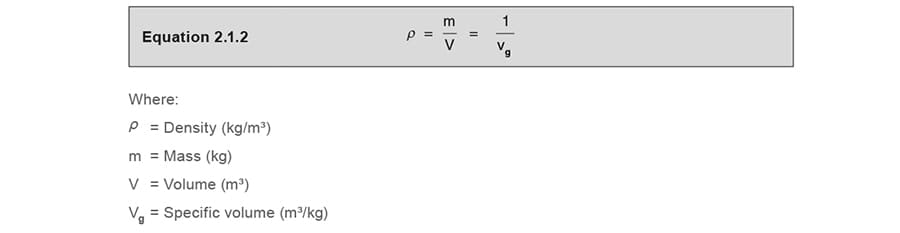The SI units of density (ρ ) are kg/m³, conversely, the units of specific volume (vg) are m³/kg.

Another term used as a measure of density is specific gravity. It is a ratio of the density of a substance (ρs) and the density of pure water (ρw) at standard temperature and pressure (STP).

This reference condition is usually defined as being at atmospheric pressure and 0°C. Sometimes it is said to be at 20°C or 25°C and is referred to as normal temperature and pressure (NTP).The density of water at these conditions is approximately 1 000 kg/m³. Therefore substances with a density greater than this value will have a specific gravity greater than 1, whereas substances with a density less than this will have a specific gravity of less than 1.

Since specific gravity is a ratio of two densities, it is a dimensionless variable and has no units. Therefore in this case the term specific does not indicate it is a property of a unit mass of a substance. Specific gravity is also sometimes known as the relative density of a substance.

Heat, work and energy

Energy is sometimes described as the ability to do work. The transfer of energy by means of mechanical motion is called work. The SI unit for work and energy is the joule, defined as 1 N m.

The amount of mechanical work carried out can be determined by an equation derived from Newtonian mechanics:

Work = Force x Displacement

It can also be described as the product of the applied pressure and the displaced volume:

Work = Applied pressure x Displaced volume

Example 2.1.1

An applied pressure of 1 Pa (or 1 N/m²) displaces a volume of 1 m³. How much work has been done?

Work done = 1 N/m² x 1 m³ = 1 N m (or 1 J)

The benefits of using SI units, as in the above example, is that the units in the equation actually cancel out to give the units of the product.

The experimental observations of J. P. Joule established that there is an equivalence between mechanical energy (or work) and heat. He found that the same amount of energy was required to produce the same temperature rise in a specific mass of water, regardless of whether the energy was supplied as heat or work.

The total energy of a system is composed of the internal, potential and kinetic energy. The temperature of a substance is directly related to its internal energy (ug). The internal energy is associated with the motion, interaction and bonding of the molecules within a substance. The external energy of a substance is associated with its velocity and location, and is the sum of its potential and kinetic energy.

The transfer of energy as a result of the difference in temperature alone is referred to as heat flow. The watt, which is the SI unit of power, can be defined as 1 J/s of heat flow.

Other units used to quantify heat energy are the British Thermal Unit (Btu: the amount of heat to raise 1 lb of water by 1 °F) and the kilocalorie (the amount of heat to raise 1 kg of water by 1 °C).

Conversion factors are readily available from numerous sources.

Specific enthalpy

This is the term given to the total energy, due to both pressure and temperature, of a fluid (such as water or steam) at any given time and condition. More specifically it is the sum of the internal energy and the work done by an applied pressure (as in Example 2.1.1).

The basic unit of measurement is the joule (J). Since one joule represents a very small amount of energy, it is usual to use kilojoules (kJ = 1 000 joules).

The specific enthalpy is a measure of the total energy of a unit mass, and its units are usually kJ/kg.

Specific heat capacity

The enthalpy of a fluid is a function of its temperature and pressure. The temperature dependence of the enthalpy can be found by measuring the rise in temperature caused by the flow of heat at constant pressure. The constant-pressure heat capacity cP, is a measure of the change in enthalpy at a particular temperature.

Similarly, the internal energy is a function of temperature and specific volume. The constant volume heat capacity cv, is a measure of the change in internal energy at a particular temperature and constant volume.

Because the specific volumes of solids and liquids are generally smaller, then unless the pressure is extremely high, the work done by an applied pressure can be neglected. Therefore, if the enthalpy can be represented by the internal energy component alone, the constant-volume and constant-pressure heat capacities can be said to be equal.

Therefore, for solids and liquids: cP ≈ cv

Another simplification for solids and liquids assumes that they are incompressible, so that their volume is only a function of temperature. This implies that for incompressible fluids the enthalpy and the heat capacity are also only functions of temperature.

The specific heat capacity represents the amount of energy required to raise 1 kg by 1 °C, and can be thought of as the ability of a substance to absorb heat. Therefore the SI units of specific heat capacity are kJ/kg K (kJ/kg °C). Water has a large specific heat capacity (4.19 kJ/kg °C) compared with many fluids, which is why both water and steam are considered to be good carriers of heat.

The amount of heat energy required to raise the temperature of a substance can be determined from Equation 2.1.4.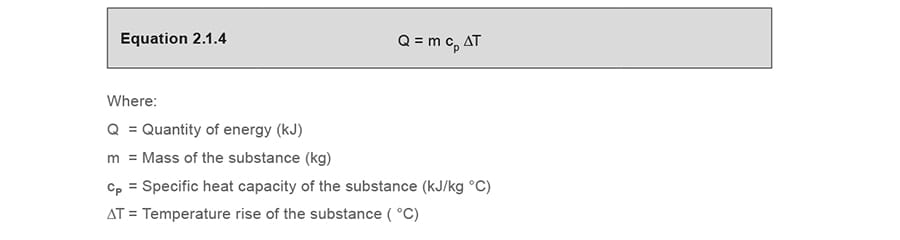This equation shows that for a given mass of substance, the temperature rise is linearly related to the amount of heat provided, assuming that the
specific heat capacity is constant over that temperature range.

Example 2.1.2

Consider a quantity of water with a volume of 2 litres, raised from a temperature of 20 °C to 70 °C.

At atmospheric pressure, the density of water is approximately 1 000 kg/m³. As there are 1 000 litres in 1 m³, then the density can be expressed as 1 kg per litre (1 kg/l). Therefore the mass of the water is 2 kg.

The specific heat capacity for water can be taken as 4.19 kJ/kg °C over low ranges of temperature.

Therefore: Q =2 kg x 4.19 kJ/kg °C x (70 - 20) °C = 419 kJ

If the water was then cooled to its original temperature of 20 °C, it would also release this amount of energy in the cooling application.

Entropy (S)

Entropy is a measure of the degree of disorder within a system. The greater the degree of disorder, the higher the entropy. The SI units of entropy are kJ/kg K (kJ/kg °C).

In a solid, the molecules of a substance arrange themselves in an orderly structure. As the substance changes from a solid to a liquid, or from a liquid to a gas, the arrangement of the molecules becomes more disordered as they begin to move more freely. For any given substance the entropy in the gas phase is greater than that of the liquid phase, and the entropy in the liquid phase is more than in the solid phase.

One characteristic of all natural or spontaneous processes is that they proceed towards a state of equilibrium. This can be seen in the second law of thermodynamics, which states that heat cannot pass from a colder to a warmer body.

A change in the entropy of a system is caused by a change in its heat content, where the change of entropy is equal to the heat change divided by the average absolute temperature, Equation 2.1.5.To look at this in further detail, consider the following examples:

Example 2.1.3

A process raises 1 kg of water from 0 to 100°C (273 to 373 K) under atmospheric conditions.

Specific enthalpy at 0°C (hf) = 0 kJ/kg (from steam tables)

Specific enthalpy of water at 100°C (hf) = 419 kJ/kg (from steam tables)

Calculate the change in specific entropy

Since this is a change in specific entropy of water, the symbol ‘s’ in Equation 2.1.6 takes the suffix ‘f’ to become sf.Example 2.1.4

A process changes 1 kg of water at 100°C (373 K) to saturated steam at 100°C (373 K) under atmospheric conditions.

Calculate the change in specific entropy of evaporation

Since this is the entropy involved in the change of state, the symbol ‘s’ in Equation 2.1.6 takes the suffix ‘fg’ to become sfg.

Specific enthalpy of evaporation

of steam at 100°C (373 K) (hfg) = 2 258 kJ/kg (from steam tables)

Specific enthalpy of evaporation

of water at 100°C (373 K) (hfg) = 0 kJ/kg (from steam tables)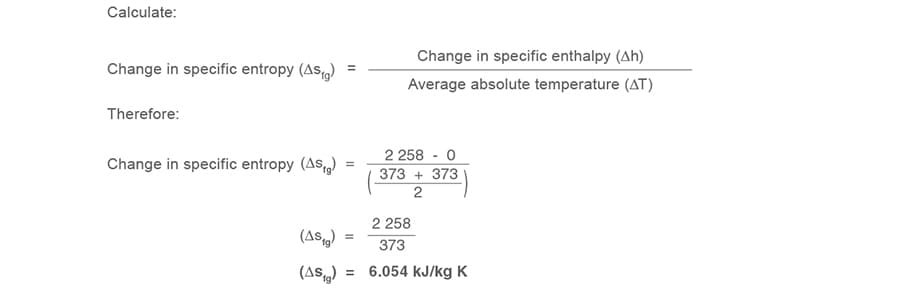The total change in specific entropy from water at 0 °C to saturated steam at 100 °C is the sum of the change in specific entropy for the water, plus the change of specific entropy for the steam, and takes the suffix ‘g’ to become the total change in specific entropy sg.

ThereforeExample 2.1.5

A process superheats 1 kg of saturated steam at atmospheric pressure to 150°C (423 K). Determine the change in entropy.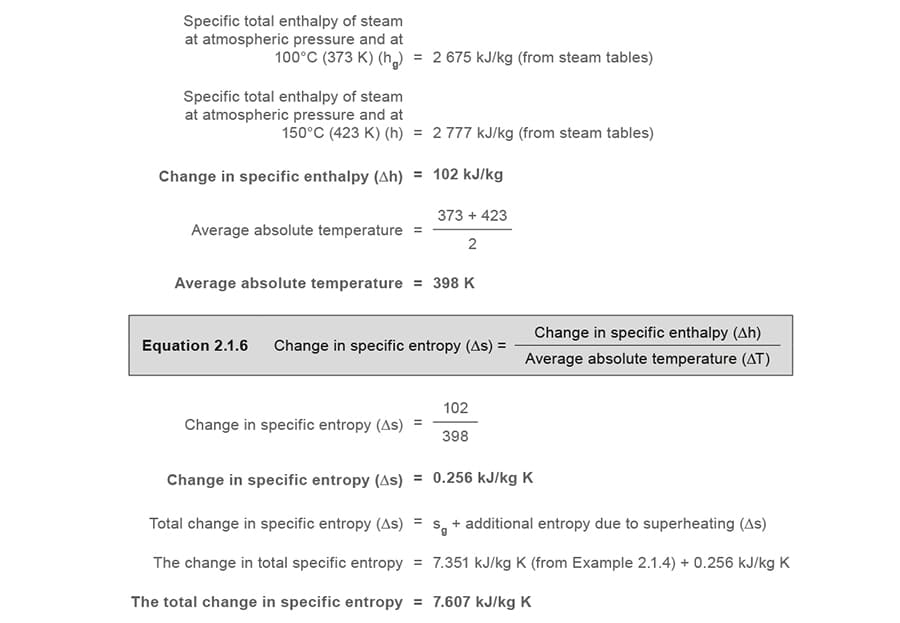As the entropy of saturated water is measured from a datum of 0.01 °C, the entropy of water at 0 °C can, for practical purposes, be taken as zero. The total change in specific entropy in this example is based on an initial water temperature of 0 °C, and therefore the final result happens to be very much the same as the specific entropy of steam that would be observed in steam tables at the final condition of steam at atmospheric pressure and 150 °C.

Entropy is discussed in greater detail in Module 2.15, Entropy - A Basic Understanding, and in Module 2.16, Entropy - Its Practical Use.